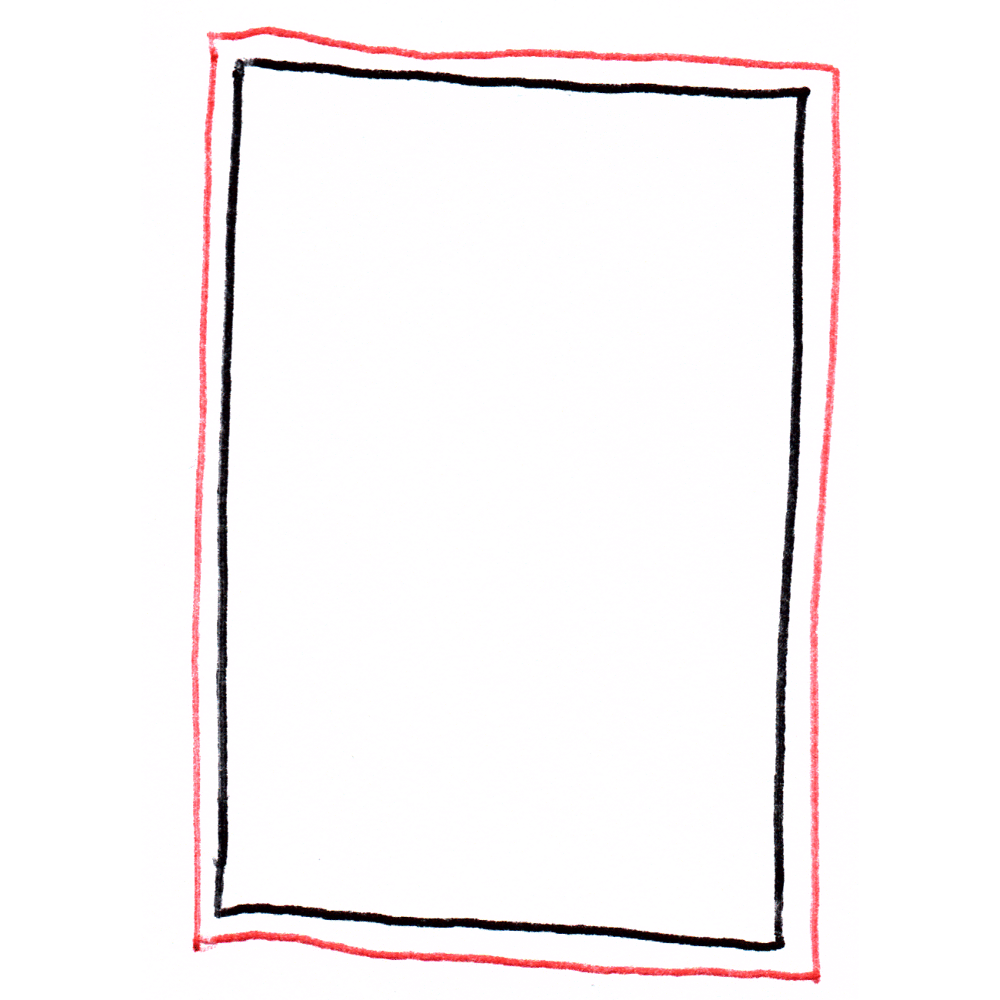# The Cartoonist's Calculator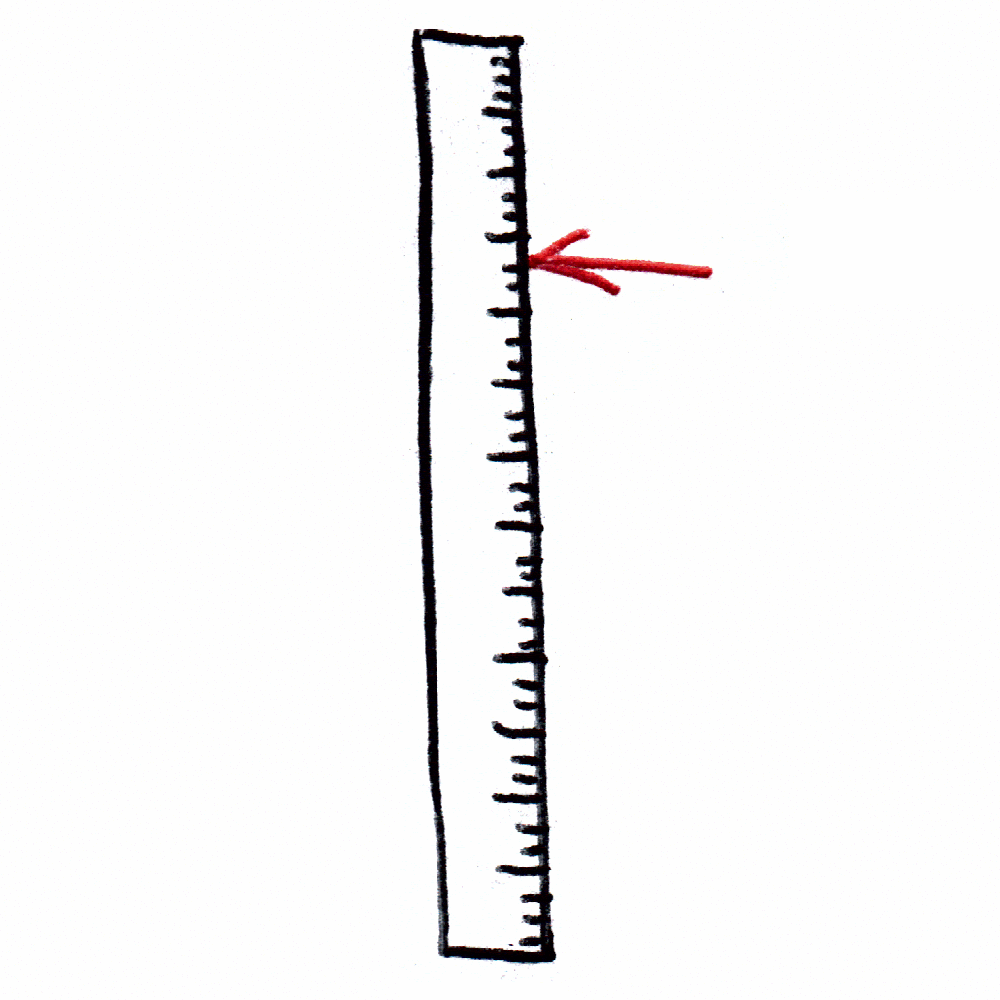## Inch Ruler Visualizer

Tip: Click red-underlined numbers to visualize them on the ruler. Try this one: 16.4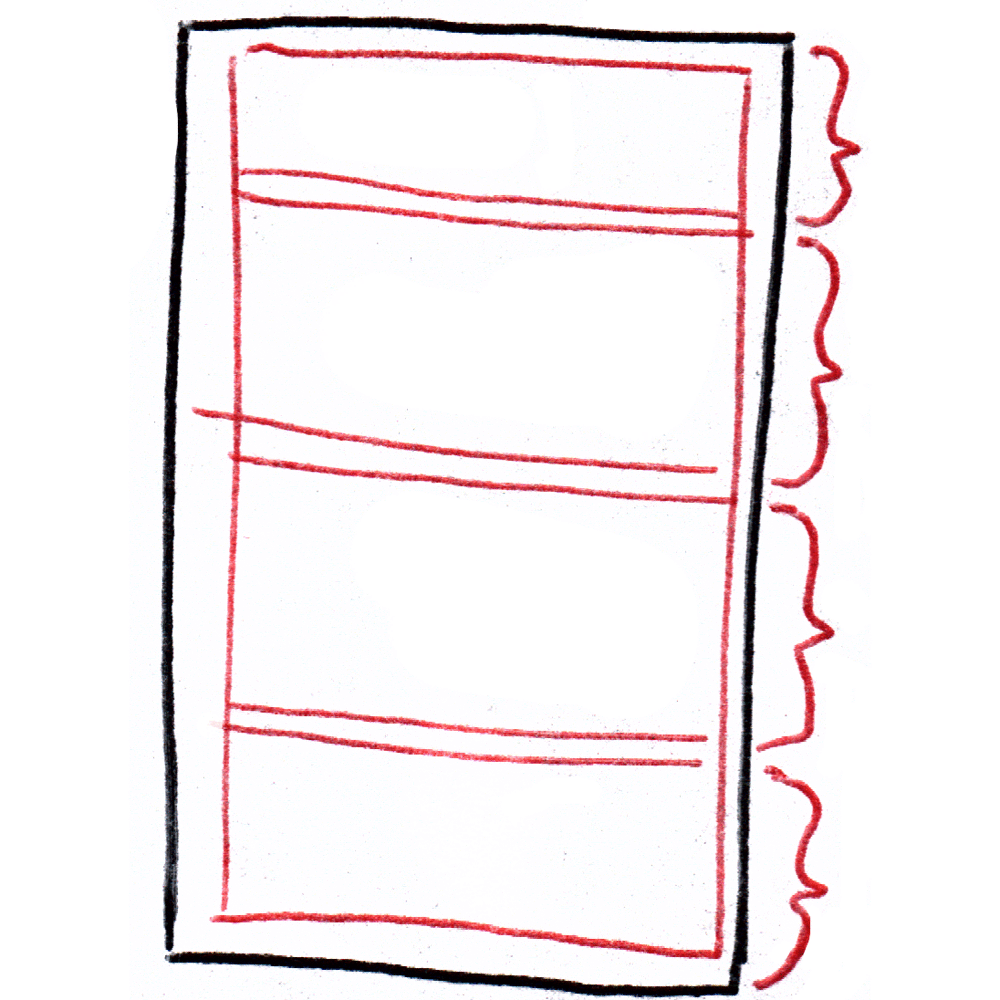## Equal panel split calculator

Divide up a tier or page into equally-sized sections, taking gutter width into account.

For example, to find the height of four equal-height tiers, on a page 12 inches high, with .25 inch gutters, you would enter: 12, 4, 0.25

Section length: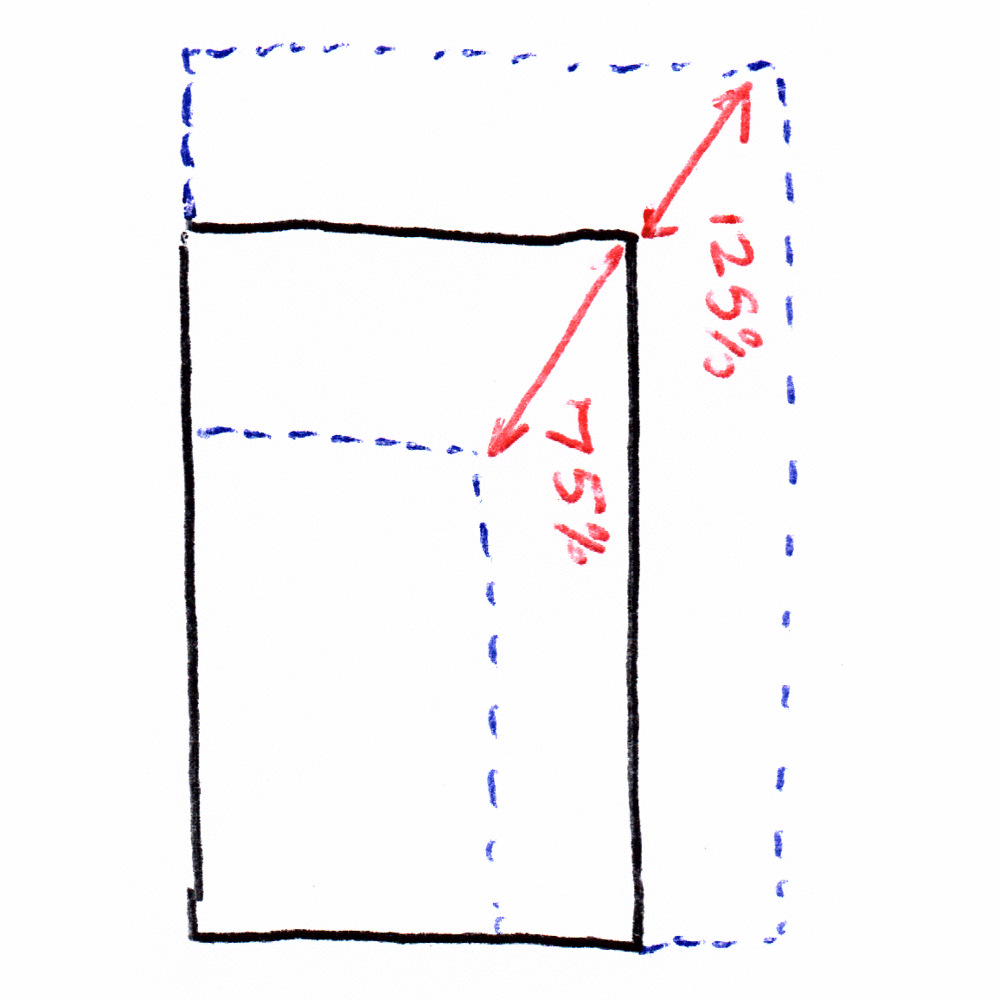## Resize by Percentage

Resized Width =
Resized Height =
To reverse this operation, scale to xx%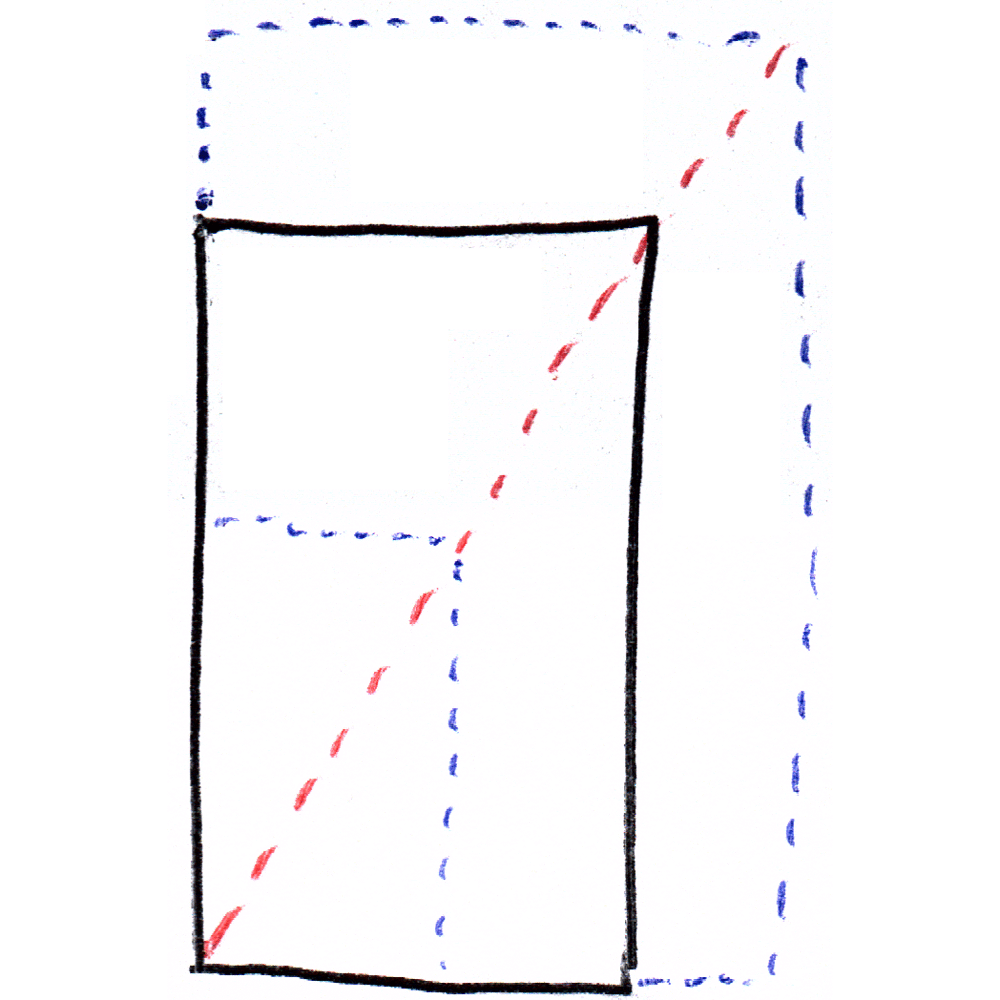## Resize Page

Calculate enlargement or reduction sizes.

New dimensions: 17 x 22.

The aspect ratio is x

This is a 200% change.

To reverse this operation, scale to xx%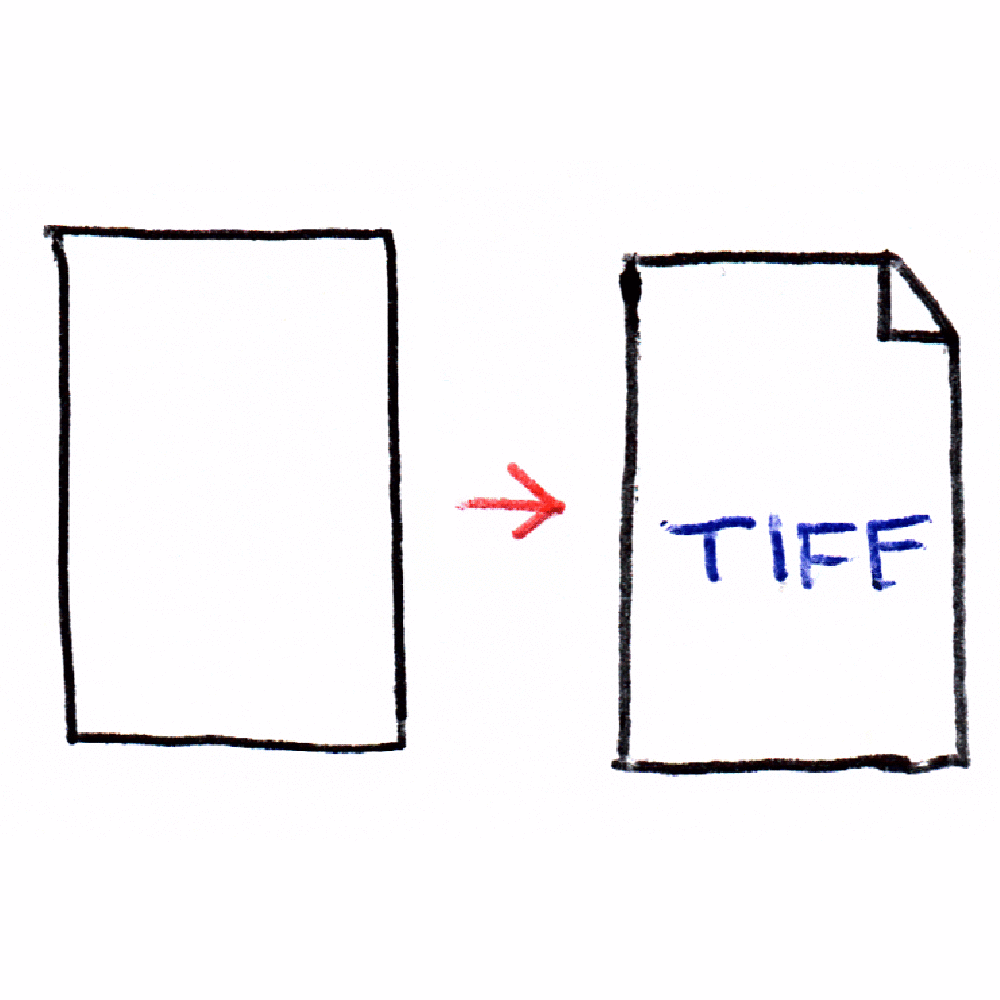## DPI Calculator (Inches to Pixels)

Calculate the digital dimensions for an image of a certain size.

Input Units:

Pixel dimensions are 4000 x 22000.

This is a 10 megapixel image.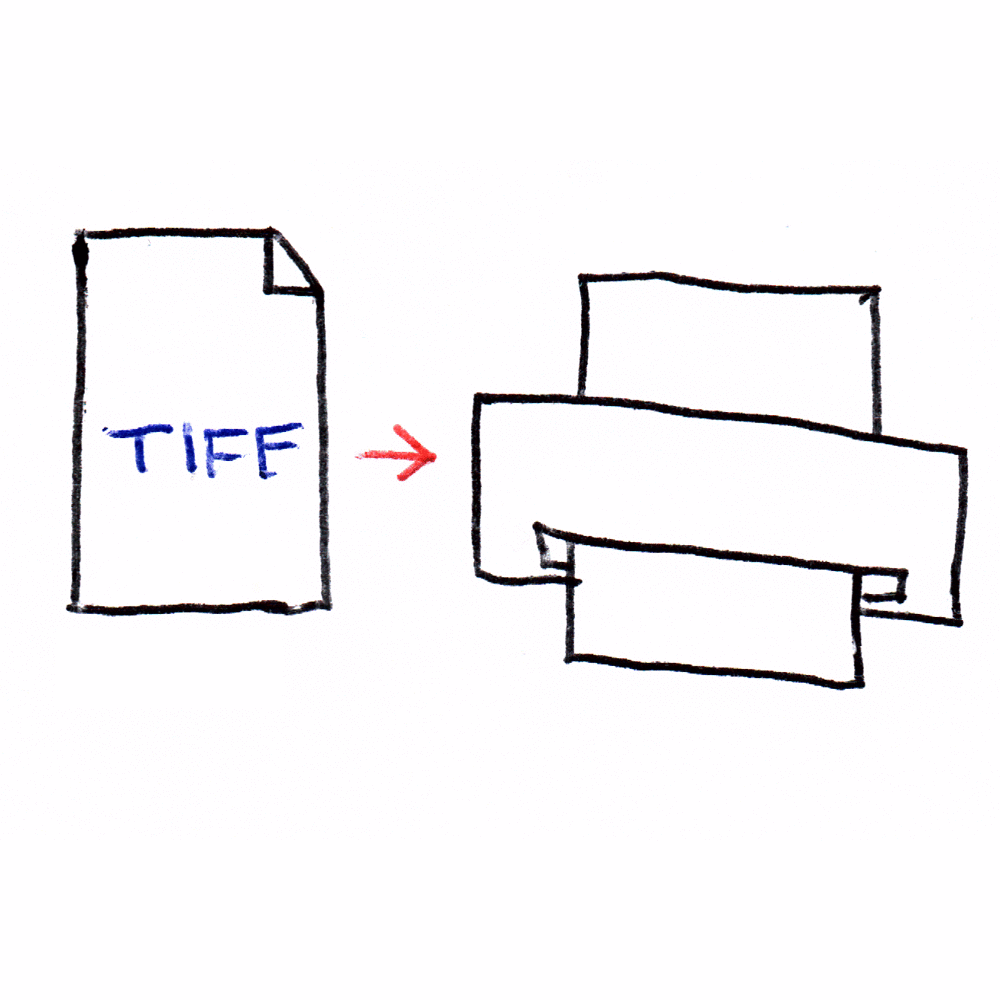## Print Size Calculator (Pixels to Inches)

Calculate the sizes a digital image can be reproduced at.

Output units:

At 100 DPI, this image will reproduce at 8.5in × 11in.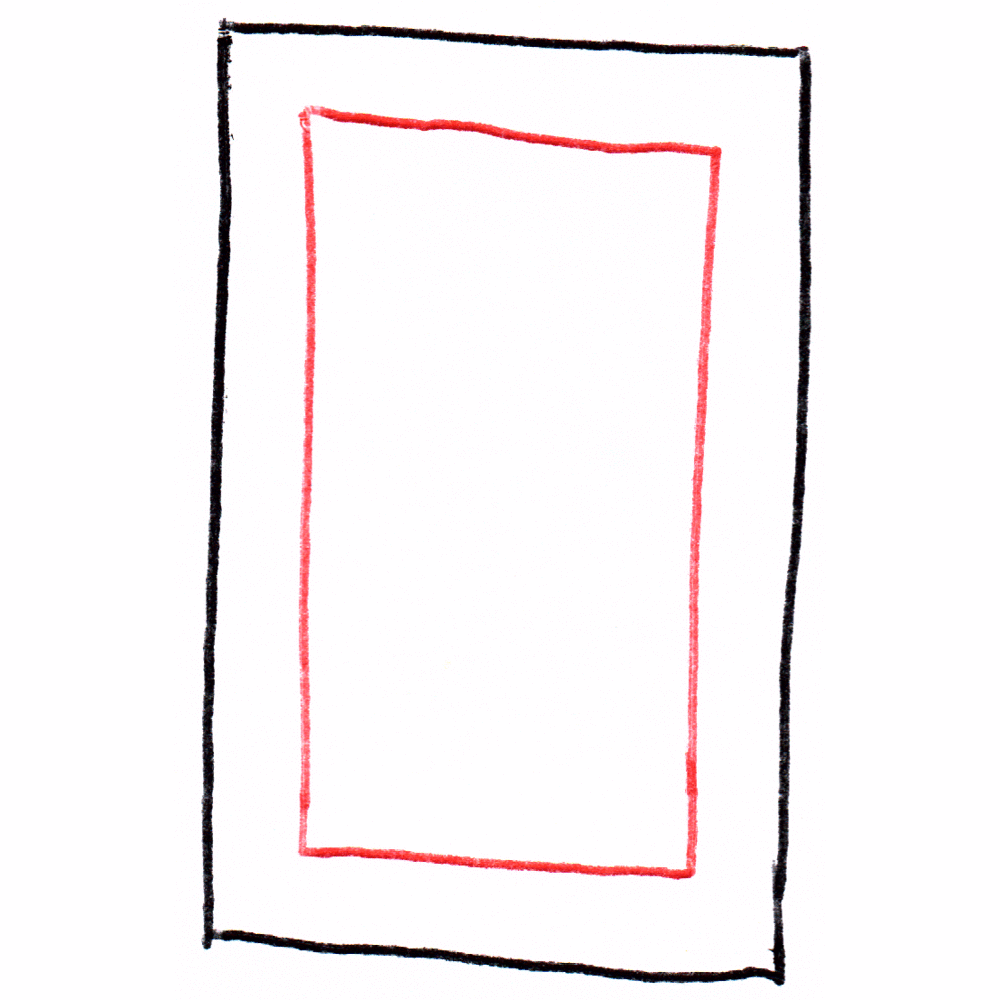## Live Area Calculator

Calculate the "live area" of a page (page size - margin).